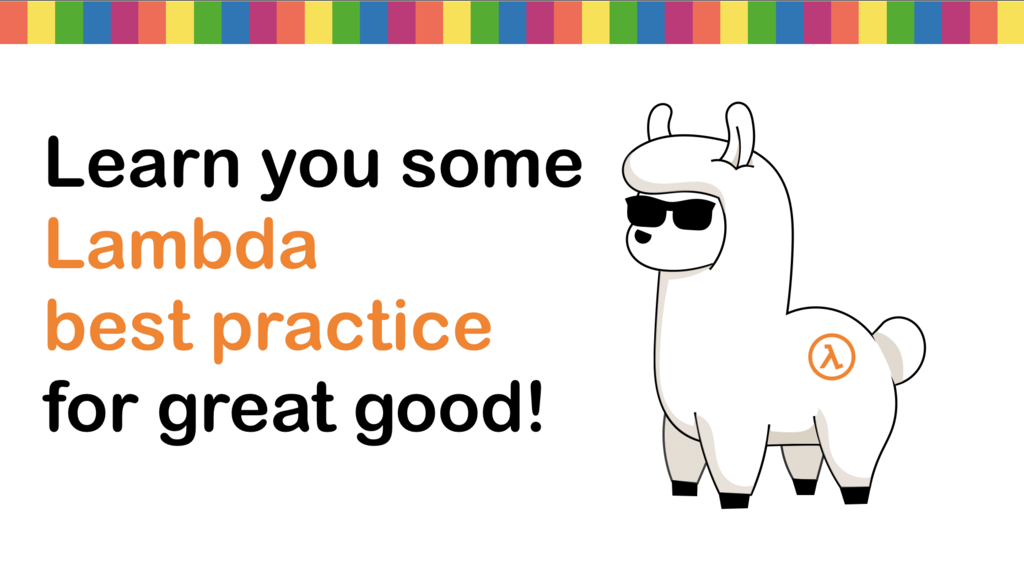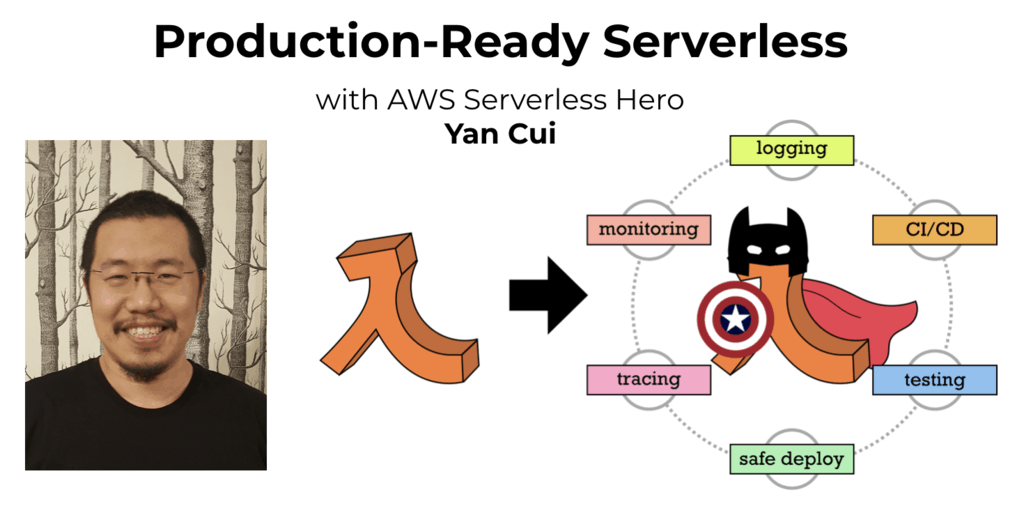# Project Euler – Problem 112 Solution

Check out my new course Learn you some Lambda best practice for great good! and learn the best practices for performance, cost, security, resilience, observability and scalability.

#### Problem

Working from left-to-right if no digit is exceeded by the digit to its left it is called an increasing number; for example, 134468.

Similarly if no digit is exceeded by the digit to its right it is called a decreasing number; for example, 66420.

We shall call a positive integer that is neither increasing nor decreasing a “bouncy” number; for example, 155349.

Clearly there cannot be any bouncy numbers below one-hundred, but just over half of the numbers below one-thousand (525) are bouncy. In fact, the least number for which the proportion of bouncy numbers first reaches 50% is 538.

Surprisingly, bouncy numbers become more and more common and by the time we reach 21780 the proportion of bouncy numbers is equal to 90%.

Find the least number for which the proportion of bouncy numbers is exactly 99%.

#### Solution

// define function f which compares the sorted and original digits of the supplied
// number n and returns if they’re the same
let f (sort:int[] -> int[]) (n:int) =
let digits = n.ToString().ToCharArray() |> Array.map (fun c -> int(c.ToString()))
not(digits |> Seq.exists2 (fun e e’ -> e <> e’) (sort digits))

// curry the function f to form the isIncreasing and isDecreasing functions
let isIncreasing = f (fun l -> Array.sort l)
let isDecreasing = f (fun l -> Array.sortBy (fun x -> 1 – x) l)

let isBouncy n = not(isIncreasing n) && not(isDecreasing n)

let mutable bouncyCount, bouncyRatio, n = 0, 0.0, 100
while bouncyRatio <> 0.99 do
n <- n+1 if isBouncy n then bouncyCount <- bouncyCount+1 bouncyRatio <- double(bouncyCount)/double(n) n [/code]Hi, I’m Yan. I’m an AWS Serverless Hero and the author of Production-Ready Serverless.

I specialise in rapidly transitioning teams to serverless and building production-ready services on AWS.

Are you struggling with serverless or need guidance on best practices? Do you want someone to review your architecture and help you avoid costly mistakes down the line? Whatever the case, I’m here to help.Check out my new course, Learn you some Lambda best practice for great good! In this course, you will learn best practices for working with AWS Lambda in terms of performance, cost, security, scalability, resilience and observability. Enrol now and enjoy a special preorder price of £9.99 (~\$13).Are you working with Serverless and looking for expert training to level-up your skills? Or are you looking for a solid foundation to start from? Look no further, register for my Production-Ready Serverless workshop to learn how to build production-grade Serverless applications!